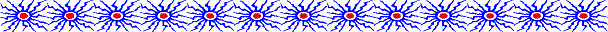# The Injected Electrons

Before we can calculate the hard x-ray emission from our loop model, we must first specify how the accelerated electrons are distributed in energy. That is, we need to specify what energies the electrons have and how many electrons are at each energy. Experience with hard x-ray spectra from flares has shown that the number of electrons per unit energy decreases with increasing energy. The number of 300 keV electrons is typically many orders of magnitude (many factors of 10) smaller than the number of 30 keV electrons.

Flare hard x-ray spectra as well as the electron distributions responsible for them are usually well described by a power law. If E represents the electron energy, the power law states that the number of electrons at energy E is proportional to E-p, where p, the power-law index, is a positive number. For p equal to 5, the value we adopt here, the ratio of the number of electrons at 30 keV to the number of electrons at 300 keV is (300 keV/30 keV)5. Therefore, the number of electrons at 30 keV is 100000 ( 105 ) times greater than the number of electrons at 300 keV.

Mathematically, our power law indicates that there is an infinite number of electrons with zero energy. Since this cannot be true physically, there must be some deviation from such a power law at low energies. For our model we assume a sharp low-energy cutoff at 30 keV. That is, we assume that no electrons are injected with energies below 30 keV.

We also need to specify the density of the injected electrons. We take the total number density (summed over all electron energies) to be 2 x 108 cm-3.

For this electron distribution we can calculate that 1.6 x 1035 electrons are injected into the cusp every second. The corresponding energy flux of injected electrons is 1.1 x 1028 ergs s-1.